# Condition for steady Precession of Heavy Symmetrical top with one point fixed

Consider the motion of a heavy symmetrical top spinning about the axis of symmetry, viz z'axis. The top is subject to a gravitational torque and is locked at its lower tip. The top's center of gravity G is located at a distance of l from its tip (O).

Since the body is symmetrical , the K.E can be written as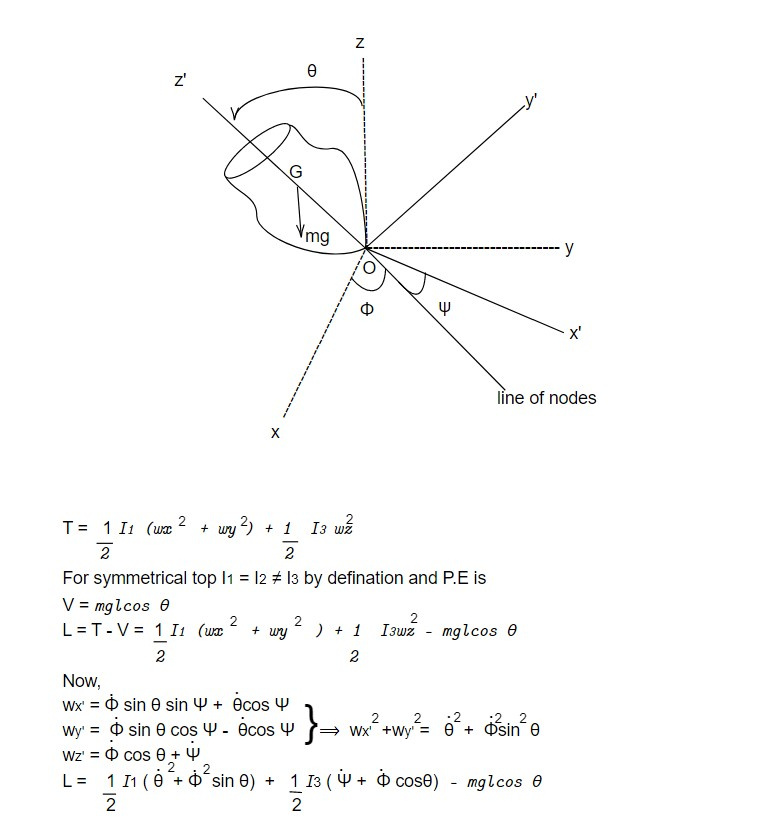Since the Lagrangian doesn't contain the Euler's angle ψ and Φ and time t, .i.e they are cyclic, the corresponding momenta pψ , pΦ and total energy E are constant in time.

We have 3 first integrals of the motion.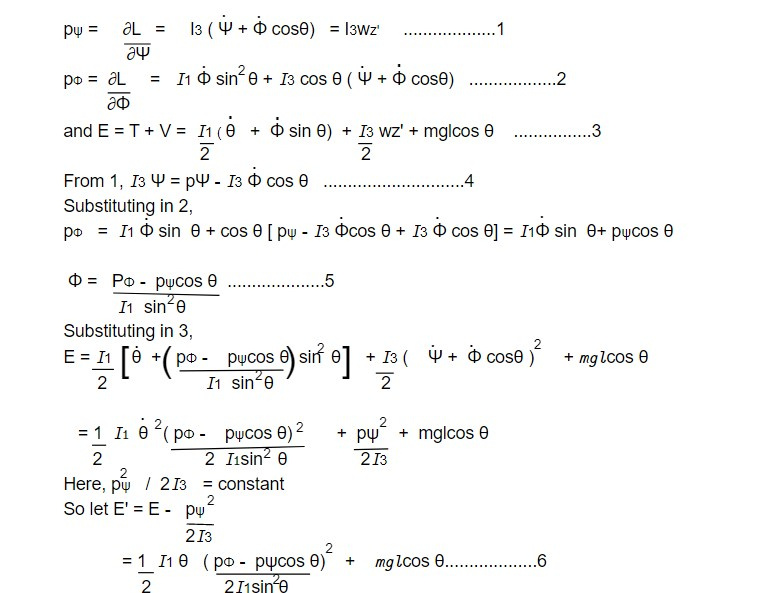When this equation is solved for as a function of t, elliptic integrals are used. Therefore, it is necessary to identify more approaches that qualitatively characterize the phenomenon. Equation 6's effective plot, which, like the pot V(θ), is a function of θ alone, is one such method: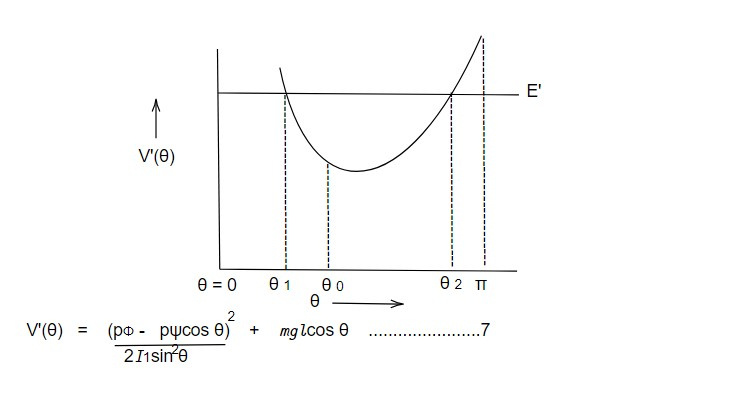Consider the rotation of effective P.E. V'(θ) with θ. It ranges between its value at θ = 0 and θ = π and tends to infinity at the end values as seen in the fig. In fig, θo at which V' is minimum must therefore be a solution of the equation: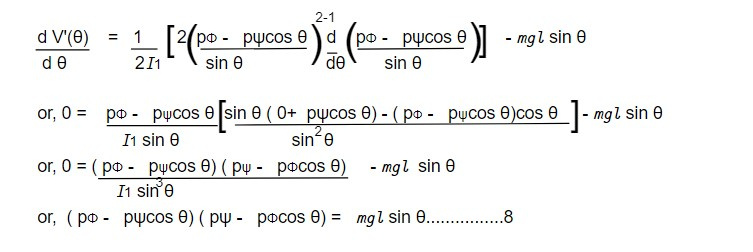E = V'(θ)

For a particular value of energy E', θ motion is a bound motion confined between the two values θ1 and θ2 of θ, are the roots of the equation.

E ' = V'(θ).......................9

The variation in the angle θ is referred to as the nutation of the symmetry axis of the top and is an up and down of the symmetry axis. If however, the minimum effective potential equals the energy E', the angle θ keeps fixed at value θo and the top precesses with the constant angle velocity is given by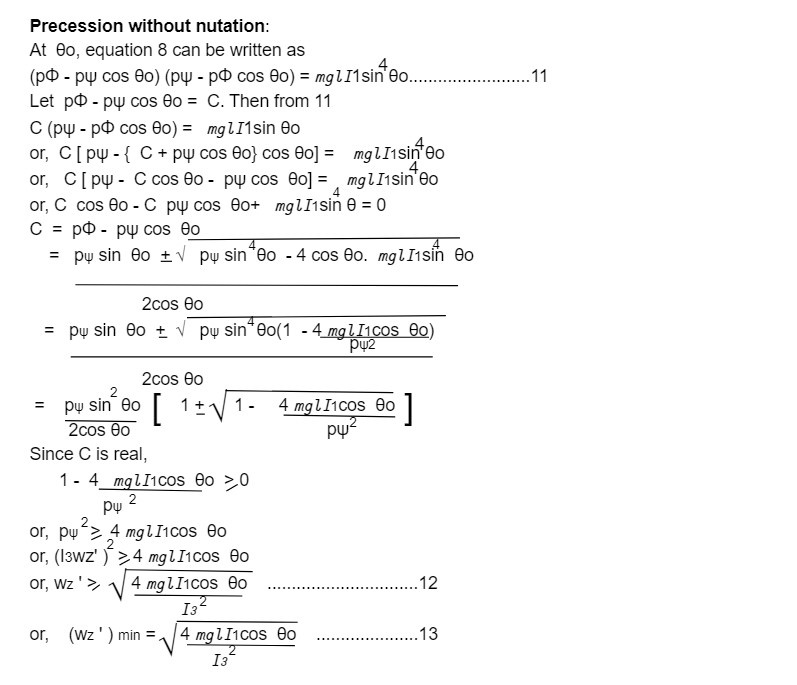Equation 13 provides the minimal value of the spin that must be present for the top to have pure steady precession; below this number, the top cannot precess uniformly. Equation 13 is also referred to as the condition for precession without nutation.

This note taken from Classical mechanics, MSC physics, Nepal.

This note is a part of the Physics Repository.

Previous topic: Heavy symmetrical top with one point fixed